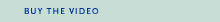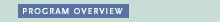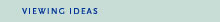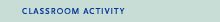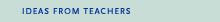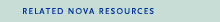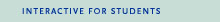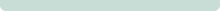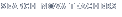Back to Teachers HomeProof, TheProgram OverviewThe Pythagorean Theorem is simple: x2 + y2 = z2. In this form, the equation can be solved. But what if the 2 is replaced with any positive integer greater than 2? Would the equation still be solvable? More than 300 years ago, amateur mathematician Pierre de Fermat said no, and claimed he could prove it. Unfortunately, the book margin in which he left this prophecy was too small to contain his thinking. Fermat's Last Theorem has since baffled mathematicians armed with the most advanced calculators and computers. NOVA chronicles the seven-year effort of one mathematician, Andrew Wiles, who methodically works in near isolation to determine the proof for this seemingly simple equation.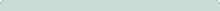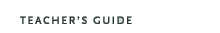Proof, TheOriginal broadcast:October 28, 1997